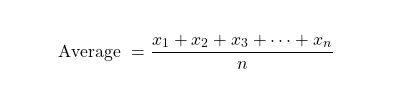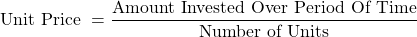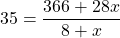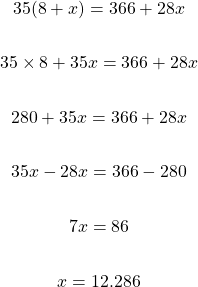# Math Calculation Examples On Unit Cost

in LeoFinance7 months ago (edited)

Hi there. In this post, I cover some unit cost math examples related to investing, stock & crypto purchases. I also cover a dollar cost averaging example.

Math text rendered with LaTeX (lay-tech) and Quicklatex.com.Source of Pixabay Picture

## Average Formula Vs Unit Price

Before getting into examples, I think it would be a good idea to show the average formula. The usual arithmetic mean or average formula is:The values are `x_1` up to `x_n` and `n` is the number of values.

For dollar cost averaging, it is more of a unit price per asset bought. It is the amount invested divided by the number of units bought.## Examples

These examples will illustrate some calculations for dollar cost averaging. The third and final example could be useful but the algebra part may not be that straightforward.

Example One

Bobby is buying physical silver as a long term investment for the next 5 years. He bought 10 troy ounces of silver at \$32 per ounce. The price of silver dipped to \$28 so Bobby buys 30 ounces at this price. Silver drops even further to \$25. He buys 21 ounces at \$25/oz.

What is the Bobby's cost per ounce of silver?

I first display the information in a table. If you have spreadsheet software like Excel that would be great too.

Ounce of SilverPrice Per Ounce of SilverAmount Invested
10oz\$3210 x \$32 = \$320
30oz\$2830 x \$28 = \$840
21oz\$2521 x \$25 = \$525
Total: 60oz--Total Amount Invested: \$1685

The total amount invested is \$1685 with 60 ounces of silver. Doing 1685 dividing by 60 gives the unit price of about \$28.08 per ounce.

Example Two

Jenny bought 3.7 Litecoin (LTC) at \$370 per LTC. The price of LTC drops to \$120. Jenny buys more LTC at this \$120 price. She buys 2 LTC at this price. Jenny then buys 0.8 LTC at \$130. What is Jenny's unit cost for LTC?

LTC AmountPrice of 1 LTCAmount Invested
3.7\$3703.7 x 370 = \$1369
2\$1202 x 120 = \$240
0.8\$1300.8 x 130 = \$104
Total: 6.5 LTC--Total Amount Invested: \$1713

The unit cost per 1 unit of Litecoin is 1713 divided by 6.5. This unit cost is \$263.54 per LTC. I wanted to have this example to show the importance of a good buy entry. It could be hard to dollar cost average down if you buy at a top and/or run out of money to buy.

Example Three - Dollar Cost Averaging

Dollar-cost averaging is a strategy where the investor purchases assets over many purchases over a period of time. Instead of one lump sum investment of \$500, the investor could do \$100 for each month for the 5 month period. Here is a table of Jack's 5 month purchasing routine of Bitcoin Cash (BCH).

Month NumberPrice of 1 BCHAmount InvestedBCH Amount
1\$370\$1000.2703 (\$100 ÷ 370)
2\$120\$1000.8333 (\$100 ÷ 120)
3\$130\$1000.7692 (\$100 ÷ 130)
4\$130\$1000.7692
5\$130\$1000.7692
Period of 5 Months--Total Amount Invested: \$500Total: About 3.4112 BCH

Jack's unit cost for 1 BCH is the amount invested divided by the amount of Bitcoin Cash. This is \$500 divided by 3.4112 BCH which is about \$146.58 per 1 unit of BCH. This unit cost amount is slightly higher than 120 or 130 per BCH but it is much lower than \$370 for 1 BCH.

Spreading out the payments is nice as the cost per unit of crypto is not entirely dependent on one single purchase.

It also helps that the prices of Bitcoin Cash was much more smaller than \$370 from month number 1. Dollar cost averaging does really well when prices are lower.

Example Four - Aiming For A Specific Unit Price

There are times when you make a bad timing when buying Bitcoin or any crypto. You do believe that the crypto price will go up in the long term and you want to accumulate in the short term. The goal is bring the unit cost to a certain level.

Jimmy bought 5 Polkadot (DOT) at a price of \$42 each and 3 DOT at \$52 each. After seeing the price drop to below \$30, Jimmy realizes he made a mistake with his timing. The current price of DOT is about \$28 for Jimmy. He wants to buy more into DOT as he thinks DOT will go higher than \$65 in the long term.

How much DOT should Jimmy buy at a price of \$28 to bring the unit cost per DOT down to \$35 per DOT?

Here is a table of Jimmy's situation. Denote `x` as the amount of DOT needed to purchase at a price of \$31 to obtain an average unit cost of \$35 per DOT

DOT AmountPrice of 1 DOTAmount Invested
5\$425 x \$42 = \$210
3\$523 x 52 = \$156
x\$2828x
Total Number of DOT: 8 + x--Total Amount Invested: \$366 + 28x

Recall that the unit price formula is:The unit price goal is \$35 and we have the amount invested and the number of units. We solve for `x`.Cross multiply or multiply both sides by `(8 + x)`. Afterwards, apply distributive law and solve for x.Jimmy needs about 12.286 DOT at a price of \$28 each to bring his average unit cost of DOT down to \$35. This 12.286 DOT is quite a bit compared to his previous purchases of 5 and 3 DOT at the higher prices.

With this example, the question seems simple but the setup and calculations is not that simple.

One more thing, the examples do not factor in trading and withdrawal fees.Pixabay Image - https://pixabay.com/photos/dollars-currency-money-us-dollars-499481/